Download Presentation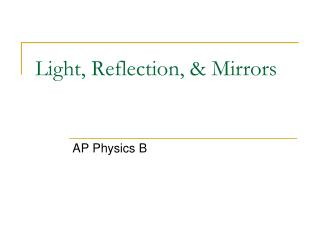Light, Reflection, & Mirrors

# Light, Reflection, & Mirrors - PowerPoint PPT Presentation

Light, Reflection, & Mirrors. AP Physics B. Facts about Light. It is a form of Electromagnetic Energy It is a part of the Electromagnetic Spectrum and the only part we can really see. Facts about Light. The speed of light, c, is constant in a vacuum. Light can be: REFLECTED ABSORBEDI am the owner, or an agent authorized to act on behalf of the owner, of the copyrighted work described.
Download Presentation## Light, Reflection, & Mirrors

An Image/Link below is provided (as is) to download presentation

Download Policy: Content on the Website is provided to you AS IS for your information and personal use and may not be sold / licensed / shared on other websites without getting consent from its author.While downloading, if for some reason you are not able to download a presentation, the publisher may have deleted the file from their server.

- - - - - - - - - - - - - - - - - - - - - - - - - - E N D - - - - - - - - - - - - - - - - - - - - - - - - - -
Presentation Transcript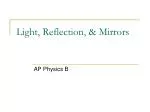### Light, Reflection, & Mirrors

AP Physics B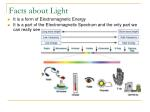• It is a form of Electromagnetic Energy
• It is a part of the Electromagnetic Spectrum and the only part we can really see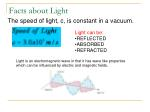The speed of light, c, is constant in a vacuum.

• Light can be:
• REFLECTED
• ABSORBED
• REFRACTED

Light is an electromagnetic wave in that it has wave like properties which can be influenced by electric and magnetic fields.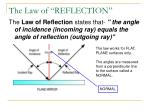The Law of “REFLECTION”

The Law of Reflection states that- " the angle of incidence (incoming ray) equals the angle of reflection (outgoing ray)"

The law works for FLAT, PLANE surfaces only.

The angles are measured from a perpendicular line to the surface called a NORMAL.

NORMAL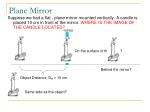Plane Mirror

Suppose we had a flat , plane mirror mounted vertically. A candle is placed 10 cm in front of the mirror. WHERE IS THE IMAGE OF THE CANDLE LOCATED?

mirror

On the surface of the mirror?

Behind the mirror?

Object Distance, Do = 10 cm

Same side as the object?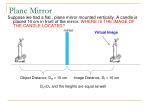Plane Mirror

Suppose we had a flat , plane mirror mounted vertically. A candle is placed 10 cm in front of the mirror. WHERE IS THE IMAGE OF THE CANDLE LOCATED?

mirror

Virtual Image

Object Distance, Do = 10 cm

Image Distance, Di = 10 cm

Do=Di, and the heights are equal as well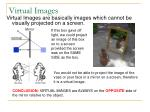Virtual Images

Virtual Images are basically images which cannot be visually projected on a screen.

If this box gave off light, we could project an image of this box on to a screen provided the screen was on the SAME SIDE as the box.

You would not be able to project the image of the vase or your face in a mirror on a screen, therefore it is a virtual image.

CONCLUSION: VIRTUAL IMAGES are ALWAYS on the OPPOSITE side of the mirror relative to the object.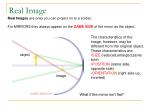Real Image

Real Images are ones you can project on to a screen.

For MIRRORS they always appear on the SAME SIDE of the mirror as the object.

• The characteristics of the image, however, may be different from the original object. These characteristics are:
• SIZE (reduced,enlarged,same size)
• POSITION (same side, opposite side)
• ORIENTATION (right side up, inverted)

object

image

What if the mirror isn’t flat?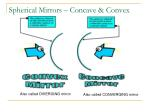Spherical Mirrors – Concave & Convex

Also called DIVERGING mirror

Also called CONVERGING mirror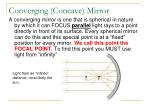Converging (Concave) Mirror

A converging mirror is one that is spherical in nature by which it can FOCUS parallel light rays to a point directly in front of its surface. Every spherical mirror can do this and this special point is at a “fixed” position for every mirror. We call this point the FOCAL POINT. To find this point you MUST use light from “infinity”

Light from an “infinite” distance, most likely the sun.Converging (Concave) Mirror

Since the mirror is spherical it technically has a CENTER OF CURVATURE, C. The focal point happens to be HALF this distance.

We also draw a line through the center of the mirror and call it the PRINCIPAL AXIS.Ray Diagram

A ray diagram is a pictorial representation of how the light travels to form an image and can tell you the characteristics of the image.

C

object

f

Principal axis

Rule One: Draw a ray, starting from the top of the object, parallel to the principal axis and then through “f” after reflection.Ray Diagrams

C

object

f

Principal axis

Rule Two: Draw a ray, starting from the top of the object, through the focal point, then parallel to the principal axis after reflection.Ray Diagrams

C

object

f

Principal axis

Rule Three: Draw a ray, starting from the top of the object, through C, then back upon itself.

THEY INTERSECT

What do you notice about the three lines?

The intersection is the location of the image.Ray Diagram – Image Characteristics

C

object

f

Principal axis

• After getting the intersection, draw an arrow down from the principal axis to the point of intersection. Then ask yourself these questions:
• Is the image on the SAME or OPPOSITE side of the mirror as the object?
• Same, therefore it is a REAL IMAGE.
• Is the image ENLARGED or REDUCED?
• Is the image INVERTED or RIGHT SIDE UP?The Mirror/Lens Equation

Is there any OTHER way to predict image characteristics besides the ray diagram? YES!

One way is to use the MIRROR/LENS equation to CALCULATE the position of the image.Mirror/Lens Equation

Assume that a certain concave spherical mirror has a focal length of 10.0 cm. Locate the image for an object distance of 25 cm and describe the image’s characteristics.

16.67 cm

What does this tell us? First we know the image is BETWEEN “C” & “f”. Since the image distance is POSITIVE the image is a REAL IMAGE.

Real image = positive image distance

Virtual image = negative image distance

What about the size and orientation?Magnification Equation

To calculate the orientation and size of the image we use the MAGNIFICATION EQUATION.

• Here is how this works:
• If we get a POSITIVE magnification, the image is UPRIGHT.
• If we get a NEGATIVE magnification, the image is INVERTED
• If the magnification value is GREATER than 1, the image is ENLARGED.
• If the magnification value is LESS than 1, the image is REDUCED.
• If the magnification value is EQUAL to 1, the image is the SAME SIZE as the object.

Using our previous data we see that our image was INVERTED, and REDUCED.Example

Assume that a certain concave spherical mirror has a focal length of 10.0 cm. Locate the image for an object distance of 5 cm and describe the image’s characteristics.

-10 cm

Characteristics?

• VIRTUAL (opposite side)
• Enlarged
• Upright

2x